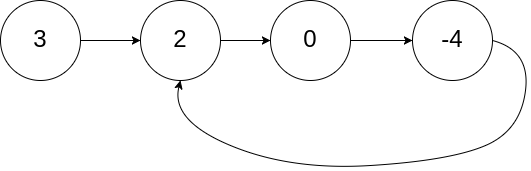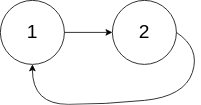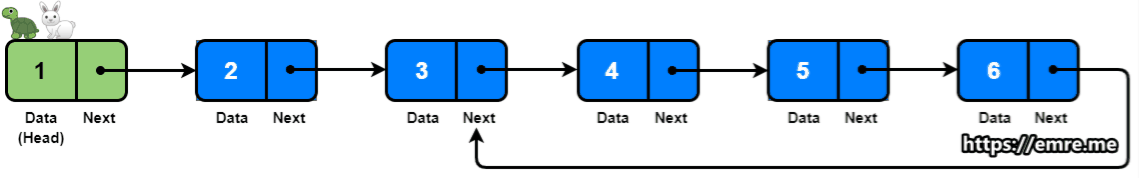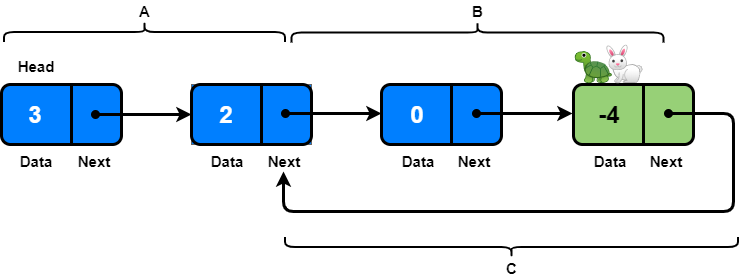# Coding Patterns: Fast & Slow Pointers

In Coding Patterns series, we will try to recognize common patterns underlying behind each algorithm question, using real examples from Leetcode.

Previous posts were about Sliding Window and Two Pointers patterns and today, we will introduce Fast & Slow Pointers pattern (a.k.a. Floyd’s Tortoise and Hare Algorithm) which is very useful when dealing with cyclic Linked Lists or Arrays.

By moving at different speeds, the algorithm proves that the two pointers are going to meet eventually. The fast pointer should catch the slow pointer once both the pointers are in a cyclic loop.

LeetCode 141 - Linked List Cycle [easy]

Given a linked list, determine if it has a cycle in it.

To represent a cycle in the given linked list, we use an integer `pos` which represents the position (0-indexed) in the linked list where tail connects to. If `pos` is -1, then there is no cycle in the linked list.

Example 1:

``````Input: head = [3, 2, 0, -4], pos = 1
Output: true
Explanation: There is a cycle in the linked list, where tail connects to
the second node.
``````Example 2:

``````Input: head = [1, 2], pos = 0
Output: true
Explanation: There is a cycle in the linked list, where tail connects to
the first node.
``````Example 3:

``````Input: head = , pos = -1
Output: false
Explanation: There is no cycle in the linked list.
``````Can you solve it using O(1) (i.e. constant) memory?

### Fast & Slow Pointers Solution

If you need to refresh your knowledge in Linked Lists, I would suggest to do so before jumping into the solution.

Imagine two racers running in a circular racing track. If one racer is faster than the other, the faster racer is bound to catch up and cross the slower racer from behind. In each iteration, Tortoise(slow pointer) moves one step and the Hare(fast pointer) moves two steps.

• If the Linked Lists does not have a cycle in it, Harewill reach the end of the Linked Lists before the Tortoiseand this will reveal that there is no cycle in the Linked Lists.
• The Tortoisewill never be catch up the Hareif there is no cycle in the Linked Lists.

If at any stage the Tortoise(slow pointer) meet with the Hare(fast pointer), we can conclude that the Linked Lists is cyclic. Here is the proof:

• If the Hare(fast pointer) is one step behind the Tortoise(slow pointer): The fast pointer moves two steps and the slow pointer moves one step, and they both meet.
• If the Hare(fast pointer) is two steps behind the Tortoise(slow pointer): The fast pointer moves two steps and the slow pointer moves one step. After the moves, the fast pointer will be one step behind the slow pointer, which reduces this scenario to the first scenario. This means that the two pointers will meet in the next iteration.``````class ListNode:
def __init__(self, val):
self.val = val
self.next = None

class Solution:
def hasCycle(self, head: ListNode) -> bool:
while fast is not None and fast.next is not None:
fast = fast.next.next
slow = slow.next
if slow == fast:
return True  # found the cycle
return False
``````

Time Complexity: O(N) where `N` is the number of nodes in the Linked Lists.

Space Complexity: O(1), algorithm runs in constant space.

## Problem: Linked List Cycle II

LeetCode 142 - Linked List Cycle II [medium]

Given a linked list, return the node where the cycle begins. If there is no cycle, return `null`.

To represent a cycle in the given linked list, we use an integer `pos` which represents the position (0-indexed) in the linked list where tail connects to. If `pos` is -1, then there is no cycle in the linked list.

Note: Do not modify the linked list.

Example 1:

``````Input: head = [3, 2, 0, -4], pos = 1
Output: tail connects to node index 1
Explanation: There is a cycle in the linked list, where tail connects to
the second node.
``````Example 2:

``````Input: head = [1, 2], pos = 0
Output: tail connects to node index 0
Explanation: There is a cycle in the linked list, where tail connects to
the first node.
``````Example 3:

``````Input: head = , pos = -1
Output: no cycle
Explanation: There is no cycle in the linked list.
``````Can you solve it without using extra space?

### Fast & Slow Pointers Solution

``````class ListNode:
def __init__(self, val):
self.val = val
self.next = None

class Solution:
def detectCycle(self, head: ListNode) -> ListNode:

while fast is not None and fast.next is not None:
fast = fast.next.next
slow = slow.next

if slow == fast:
while current is not slow:
current = current.next
slow = slow.next
return slow
return None
``````

Time Complexity: O(N) where `N` is the number of nodes in the Linked Lists.

Space Complexity: O(1), algorithm runs in constant space.

As you can see, only difference between two problems is the part:

``````if slow == fast:
while current is not slow:
current = current.next
slow = slow.next
return slow
``````

Let’s try to understand it with a diagram:When the Hare(fast pointer) and the Tortoise(slow pointer) meet at point -4, the length they have run are `A+2B+C` (for Hare) and `A+B` (for Tortoise).

Since the fast pointer is 2 times faster than the slow pointer, `A+2B+C == 2(A+B)`, then we get `A==C`.

So, when another pointer (`current`) run from `head` to 2 (distance `A`), at the same time, previous `slow` pointer will run from -4 to 2 (distance `C`), so they meet at the pointer 2 together, which is the point where cycle begins as problem asked.

## How to identify?

This approach is quite useful when dealing with cyclic Linked Lists or Arrays.

When the problem involves something related to cyclic data structures, you should think about Fast & Slow Pointers pattern.

Tags:

Categories:

Updated: